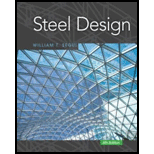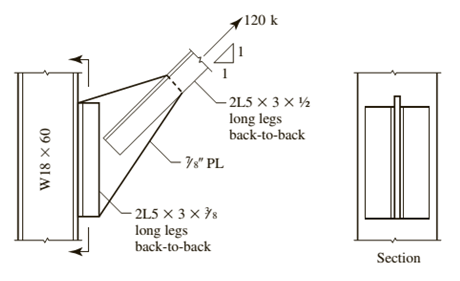# A double-angle tension member is attached to a 7 8 -inch gusset plate, which in turn is connected to a column flange via another pair of angles as shown in Figure P7.9-4. The given load is a service load consisting of 25 % dead load and 75 % live load. All connections are to be made with 7 8 -inch-diameter, Group B slip-critical bolts. Assume that the threads are in shear. Determine the required number of bolts and show their location on a sketch. The column is of A992 steel and the angles and plate are A36. a . Use LRFD. b . Use ASD.### Steel Design (Activate Learning wi...

6th Edition
Segui + 1 other
Publisher: Cengage Learning
ISBN: 9781337094740

#### Solutions

Chapter
Section### Steel Design (Activate Learning wi...

6th Edition
Segui + 1 other
Publisher: Cengage Learning
ISBN: 9781337094740
Chapter 7, Problem 7.9.4P
Textbook Problem
47 views

## A double-angle tension member is attached to a 7 8 -inch gusset plate, which in turn is connected to a column flange via another pair of angles as shown in Figure P7.9-4. The given load is a service load consisting of 25 % dead load and 75 % live load. All connections are to be made with 7 8 -inch-diameter, Group B slip-critical bolts. Assume that the threads are in shear. Determine the required number of bolts and show their location on a sketch. The column is of A992 steel and the angles and plate are A36.a. Use LRFD.b. Use ASD.To determine

(a)

The number of bolts using Load and Resistance Factor Design (LRFD).

### Explanation of Solution

Given:

The diameter of the bolt is 78inch.

Concept Used:

Write the expression for the number of bolts.

n=AboltAbolt ....... (I)

Here, the total number of bolts is Abolt, the area of the bolt is Abolt.

Write the expression for the area of the bolt.

Abolt=π4d2 ....... (II)

Here, the diameter of the bolt is d.

Write the expression for the nominal tensile stress in the presence of shear.

Fnt'=1.3FntFntϕFnvfnv ...... (III)

Here, the nominal tensile stress in the absence of shear is Fnt', the nominal tensile stress in the absence of shear is Fnt, the load reduction factor is ϕ, the nominal shear stress in the absence of shear is Fnv, the required shear stress is fnv.

Write the expression for the bearing strength of the bolt.

ϕRn=Fnt'Abolt ...... (IV)

Here, the bearing strength is Rn.

Rewrite the Equation (IV).

ϕRnAbolt=Fnt' ....... (V)

Rewrite the equation (III), by substituting Equation (V).

ϕRnAbolt=[1.3FntFntϕFnvfnv] ...... (VI)

Write the expression for the bearing strength of the bolt.

Rn=2.4dtFu ...... (VII)

Here, the ultimate shear strength is Fu.

Write the expression for the required shear stress.

fnv=VboltAbolt ...... (VIII)

Here, the shear force is Vbolt.

Write the expression for the shear force in bolt.

Vbolt=Vum ...... (IX)

Here, the factored load is Vu and the slope of line of action is m.

Write the expression for the factored load in shear.

Vu=1.2D+1.6L ...... (X)

Calculation:

The nominal tensile stress in the absence of shear is 90ksi.

The nominal shear stress in the absence of shear is 54ksi.

Calculate the area of the bolt.

Substitute 78in for d in the Equation (II).

Abolt=π4(78in)2=0.601in2

Calculate the factored load in shear.

Substitute 0.25×120ksi for D, 0.75×120ksi for L in the Equation (X)

To determine

(b)

The number of bolts using Allowed Strength Design (ASD).

### Still sussing out bartleby?

Check out a sample textbook solution.

See a sample solution

#### The Solution to Your Study Problems

Bartleby provides explanations to thousands of textbook problems written by our experts, many with advanced degrees!

Get Started

Find more solutions based on key concepts
Describe factors that affect thermal comfort.

Engineering Fundamentals: An Introduction to Engineering (MindTap Course List)

What is the benefit of using the offset tailstock method for turning tapers?

Precision Machining Technology (MindTap Course List)

What types of files might you want to transfer between your computer and mobile device using Bluetooth?

Enhanced Discovering Computers 2017 (Shelly Cashman Series) (MindTap Course List)

What does the AWS abbreviation “SENSE” stand for?

Welding: Principles and Applications (MindTap Course List)

If your motherboard supports ECC DDR3 memory, can you substitute non-ECC DDR3 memory?

A+ Guide to Hardware (Standalone Book) (MindTap Course List)